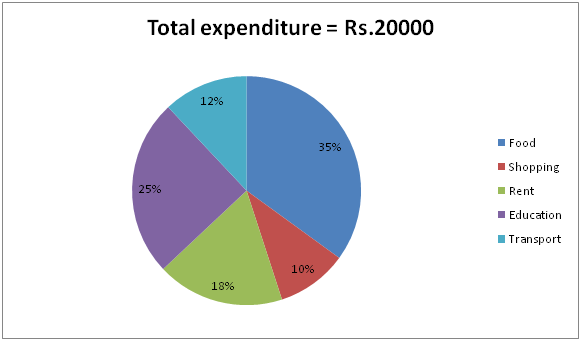# SBI Clerk Pre Quantitative Aptitude (Day-44)

Dear Aspirants, Our IBPS Guide team is providing new series of Quantitative Aptitude Questions for SBI Clerk Prelims 2020 so the aspirants can practice it on a daily basis. These questions are framed by our skilled experts after understanding your needs thoroughly. Aspirants can practice these new series questions daily to familiarize with the exact exam pattern and make your preparation effective.

Start Quiz

Ensure Your Ability Before the Exam – Take SBI Clerk 2020 Prelims Free Mock Test

Inequality

Directions (1 – 5): Following questions have two quantities as Quantity I and Quantity II. You have to determine the relationship between them and give answer as,

a) If quantity I > quantity II

b) If quantity I < quantity II

c) If quantity I ≥ quantity II

d) If quantity I ≤ quantity II

e) If quantity I = quantity II or no relation can be established.

1) Quantity I: What is the profit percent earned selling a product for Rs.3000, if the amount of profit earned on selling the product was Rs.1000?

Quantity II: If the selling price of the product was twice the cost price, then what is the profit percent earned while selling the product for Rs.2000?

2) Quantity I: The ratio of two numbers is 5: 4 and their difference is 2. What is the sum of these numbers?

Quantity II: The ratio of three numbers is 1: 2: 3 and the sum of their squares is 14. What is the sum?

3) Quantity I: Salaries of Raman and Velu are in the ratio 2: 5. If the salary of each is increased by Rs.2000, the new ratio becomes 4: 9. What is Velu’s salary?

Quantity II: Anu, Bala and Prem started a business in partnership by investing in the ratio 5: 4: 6 respectively. At the end of the year they earned a profit of Rs.75000. What will be Anu’s share?

4) Quantity I: Find C.P, when S.P = Rs.33 and gain = 10%?

Quantity II: Find S.P, when C.P = Rs.40 and loss = 25%?

5) Quantity I: Find the values of x, if x2 – 18x + 80 = 0.

Quantity II: Find the values of x, if x2 – 11x + 24 = 0.

Data Interpretation

Directions (6 – 10): Study the following information carefully and answer the questions given below?

The given pie chart shows the percentage amount spends on food, shopping, rent, education and transport in a family.6) What is the average amount of money spends on Rent, Food and Shopping together?

a) Rs.3600

b) Rs.4000

c) Rs.4200

d) Rs.4500

e) Rs.3800

7) If the ratio of income to savings is 5:1, then what is the savings of the family?

a) Rs.4000

b) Cannot be determine

c) Rs.3600

d) Rs.5000

e) None of these

8) If the family bought only watch and shirt in the shopping and the cost price of the watch is Rs.1200, then what is the cost price of shirt?

a) Rs.400

b) Rs.500

c) Rs.300

d) Rs.600

e) None of these

9) What is the difference between the amounts spends on education and Transport?

a) Rs.1800

b) Rs.2000

c) Rs.1500

d) Rs.2600

e) Rs.2200

10) What is the central corresponding angle of Food?

a) 1160

b) 1260

c) 1180

d) 1200

e) None of these

Directions (1-5) :

Quantity I:

S.P = Rs.3000, C.P = 3000 – 1000 = Rs.2000

Profit % = (S.P – C.P)/C.P * 100 = 1/2000 * (3000 – 2000) * 100 = 50%

Quantity II:

C.P = S.P/2 = 2000/2 = Rs.1000

Profit % = (S.P – C.P)/C.P * 100 = 1/1000 * (2000 – 1000) * 100 = 100%

Hence, Quantity I < Quantity II

Quantity I:

Let the two numbers be 5x and 4x.

5x – 4x = 2 => x = 2

Numbers are 10 and 8. Sum of these numbers = 10 + 8 = 18

Quantity II:

Let the three numbers be x, 2x and 3x.

x2 + (2x)2 + (3x)2 = 14 => x =1

Numbers are 1, 2 and 3. Sum of these numbers = 1 + 2 + 3 = 6

Hence, Quantity I > Quantity II

Quantity I:

Raman’s salary = 2x and Velu’s salary = 5x

(2x + 2000)/ (5x + 2000) = 4/9

=> x = 5000

Raman’s salary = Rs.10000 and Velu’s salary = Rs.25000

Quantity II:

Ratio of investments = 5: 4: 6

Profit = Rs.75000

Anu’s share = 5/15 * 75000 = Rs.25000

Hence, Quantity I = Quantity II

Quantity I:

C.P = 100 * S.P/ (100 + Gain %)

= 100 * 33/ (100 + 10) = Rs.30

Quantity II:

S.P = (100 – Loss %) / 100 * C.P

= (100 – 25)/100 * 40 = Rs.30

Hence, Quantity I = Quantity II

Quantity I:

x2 – 18x + 80 = 0

=> (x – 8) (x – 10) = 0

=> x = 8, 10

Quantity II:

x2 – 11x + 24 = 0

=> (x – 8) (x – 3) = 0

=> x = 8, 3

Hence, Quantity I ≥ Quantity II

Directions (6-10) :

Required average

= (35 + 10 + 18)/(3 * 100) * 20000

= 4200

Expenditure = 5x – x = 4x

4x = 20000

x = Rs.5000

Shopping = 10/100 * 20000 = Rs.2000

CP of shirt = 2000 – 1200 = Rs.800

Difference = 25 – 12/100 * 20000 = Rs.2600